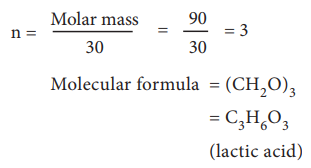Home | | Chemistry 11th std | Calculation of Molecular Formula from Empirical Formula

# Calculation of Molecular Formula from Empirical Formula

Let us understand the calculations of molecular mass from the following example.

Calculation of Molecular Formula from Empirical Formula:Let us understand the calculations of molecular mass from the following example.

Two organic compounds, one present in vinegar (molar mass: 60 g mol–1), another one present in sour milk (molar mass 90 g mol–1) have the following mass percentage composition. C-40%, H-6.6% ; O-53.4%. Find their molecular formula.

Since both compounds have same mass percentage composition, their empirical formula are the same as worked out in the example problem no 2. Empirical formula is CH2O. Calculated empirical formula mass (CH2O) = 12 + (21) + 16 = 30 g mol–1.

Formula for the compound present in vinegarCalculation of molecular formula for the compound present in sour milk.Study Material, Lecturing Notes, Assignment, Reference, Wiki description explanation, brief detail
11th Chemistry : UNIT 1 : Basic Concepts of Chemistry and Chemical Calculations : Calculation of Molecular Formula from Empirical Formula |